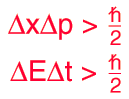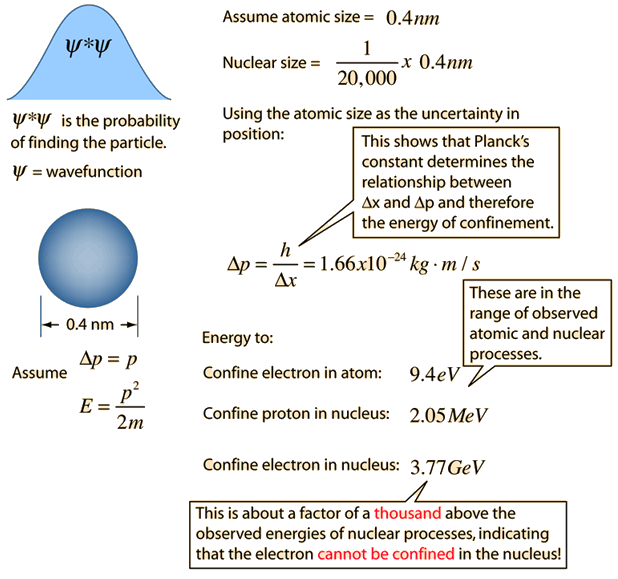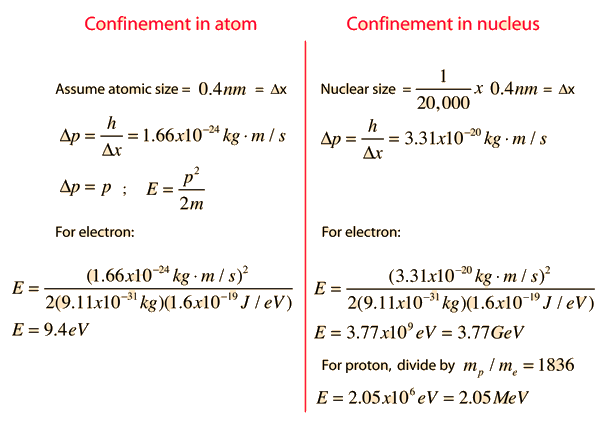# The Uncertainty Principle

The position and momentum of a particle cannot be simultaneously measured with arbitrarily high precision. There is a minimum for the product of the uncertainties of these two measurements. There is likewise a minimum for the product of the uncertainties of the energy and time.This is not a statement about the inaccuracy of measurement instruments, nor a reflection on the quality of experimental methods; it arises from the wave properties inherent in the quantum mechanical description of nature. Even with perfect instruments and technique, the uncertainty is inherent in the nature of things.

### Graphical interpretation of uncertainty principle

Index

Uncertainty principle concepts

 HyperPhysics***** Quantum Physics R Nave
Go Back

# Uncertainty Principle

Important steps on the way to understanding the uncertainty principle are wave-particle duality and the DeBroglie hypothesis. As you proceed downward in size to atomic dimensions, it is no longer valid to consider a particle like a hard sphere, because the smaller the dimension, the more wave-like it becomes. It no longer makes sense to say that you have precisely determined both the position and momentum of such a particle. When you say that the electron acts as a wave, then the wave is the quantum mechanical wavefunction and it is therefore related to the probability of finding the electron at any point in space. A perfect sinewave for the electron wave spreads that probability throughout all of space, and the "position" of the electron is completely uncertain.### Forms of uncertainty principle

 Application example: required energy to confine particles
Index

 HyperPhysics***** Quantum Physics R Nave
Go Back

# Particle Confinement

The uncertainty principle contains implications about the energy that would be required to contain a particle within a given volume. The energy required to contain particles comes from the fundamental forces, and in particular the electromagnetic force provides the attraction necessary to contain electrons within the atom, and the strong nuclear force provides the attraction necessary to contain particles within the nucleus. But Planck's constant, appearing in the uncertainty principle, determines the size of the confinement that can be produced by these forces. Another way of saying it is that the strengths of the nuclear and electromagnetic forces along with the constraint embodied in the value of Planck's constant determine the scales of the atom and the nucleus.

The following very approximate calculation serves to give an order of magnitude for the energies required to contain particles.### More detailed treatment of 3-D box

 Energies in electron volts (eV)
 Application example: required energy to confine particles
Index

 HyperPhysics***** Quantum Physics R Nave
Go Back

# Confinement CalculationIf you examine this calculation in detail, you will note that a gross approximation was made in the relationship Δp = h/Δx. This was done to get a qualitative relationship that shows the role of Planck's constant in the relationship between Δx and Δp and thus the role of h in determining the energy of confinement. The other reason for doing it was to get an electron confinement energy close to what is observed in nature for comparison with the energy for confining an electron in the nucleus. If you actually use the limiting case allowed by the uncertainty principle, Δp = hbar/2Δx, the confinement energy you get for the electron in the atom is only 0.06 eV. This is because this approach only confines the electron in one dimension, leaving it unconfined in the other directions. For a more realistic atom you would need to confine it in the other directions as well. A better approximation can be obtained from the three-dimensional particle-in-a-box approach, but to precisely calculate the confinement energy requires the Shrodinger equation (see hydrogen atom calculation).

### Calculation for 3-D box

Index

 HyperPhysics***** Quantum Physics R Nave
Go Back

# Wavefunction Contexts### Calculation for 3-D box

Index

 HyperPhysics***** Quantum Physics R Nave
Go Back